Cím: Planar point sets with strong properties of symmetry Szerző(k): Füzet: 2004/januári melléklet, 2 - 8. oldal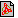PDF file Témakör(ök): Matematika

A szöveg csak Firefox böngészőben jelenik meg helyesen. Használja a fenti PDF file-ra mutató link-et a letöltésre.

1
2
One of the problems given at the final of the National Academic Competition of Secondary School Students (OKTV) 2002‐2003 was to prove the following statement (slightly rephrased):

If any three-element subset of a $2003$-element planar point set is axially symmetric, then all the points in the set are collinear.

To solve the problem did not prove to be difficult, and an abundance of correct solutions was received. It was clear from the solution of several competitors, that the proposition holds for point sets consisting of much fewer points than 2003. Moreover, someone also made a remark that the point set consisting of the vertices and the centre of a regular pentagon shows that the statement is not true for six-element point sets, and formed a conjecture that it is true for point sets of more than six elements.
We are going to prove this proposition by considering the potential point sets and finally mention some possible ways to generalize these.

3-symmetric sets

A subset of the plane is called $3$-symmetric, if any three-element subset of it is axially symmetric. These are described by the following theorem:

Theorem. A subset of the plane is $3$-symmetric if and only if it is a subset of any one of the following $3$-symmetric point sets:
 $\left(a\right)$ a straight line;
 $\left(b\right)$ the vertices of an isosceles triangle and the centre of its circumscribed circle;
 $\left(c\right)$ the four vertices of a rhombus;
 $\left(d\right)$ the four vertices and the centre of a square;
 $\left(e\right)$ the five vertices and the centre of a regular pentagon;
 $\left(f\right)$ four vertices of a regular pentagon and the intersection point of the diagonals of the received trapezium;
 $\left(g\right)$ four vertices of a regular pentagon and the intersection point of the extensions of the non-parallel sides of the received trapezium;
 $\left(h\right)$ set $\text{P}$ derived the following way: Let $AECUV$ be a regular pentagon, $D$ be the intersection of diagonals $AC$ and $EV$, $B$ be the intersection of diagonals $AC$ and $EU$, and $E\text{'}$ be the reflection of $E$ about $AC$. Then $\text{P}=\left\{A,B,C,D,E,E\text{'}\right\}$;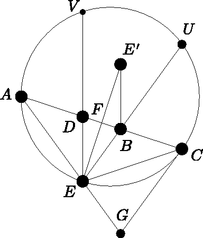Figure $\left(h\right)$

 $\left(k\right)$ set $\text{P}$ derived the following way: let $AEXYCUV$ be a regular heptagon, $B$ be the intersection of diagonals $AC$ and $EU$, and $E\text{'}$ be the reflection of $E$ about $AC$; then $\text{P}=\left\{A,B,C,E,E\text{'}\right\}$.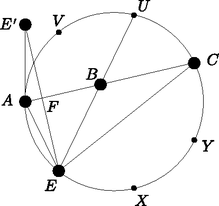Figure $\left(k\right)$

Figures $\left(h\right)$ and $\left(k\right)$ describe their corresponding configurations. However, it is worth mentioning that $ABCEU$ in the first figure is configuration $\left(f\right)$, whereas $ABCE\text{'}U$ and $ABCEG$ is configuration $\left(g\right)$, where $G$ is the reflection of $U$ about $AC$. This latter statement holds due to the fact that $E\text{'}BCU$ and $EBCG$ are both four vertices of a regular pentagon.

The theorem is proved through a series of propositions. Let $\text{P}$ be a given $3$-symmetric set, which is assumed to be non-collinear. Since all subsets of $\text{P}$ are $3$-symmetric and all configurations except $\left(a\right)$ in the theorem above have at most $6$ elements, it can be assumed (and it is indeed assumed until the end of the proof), that $\text{P}$ is finite.

Proposition 1. If $A,B,C\in \text{P}$, and angle $BAC$ is at least a right angle, but is not a straight angle, then $AB=AC$.

Proof. Triangle $ABC$ is axially symmetric and therefore it is isosceles, so there are acute angles opposite the equal sides.  $\square$

First, we are going to examine the case in which $\text{P}$ contains three collinear points. It is advisable to follow the steps of the proof in the figures above, notations having been kept.

Proposition 2. Let us suppose that points $A,B,C\in \text{P}$ lie along a straight line $e$ in this order, and $E\in \text{P}$ is an external point. Let $a=AB$, $c=CB$. Then, (after swapping $A$ and $C$ if necessary) one of the following four possibilities holds:
 $\left(1\right)$ $a=c=EB$ and $EA=EC$. In this case $A$, $E$, $C$ are three vertices of a square and $B$ is its centre;
 $\left(2\right)$ $EB=c$, $EA=EC=a$. In this case the values of angles $EAC$ and $ECA$ are $\frac{\pi }{5}$, while the value of angle $AEC$ is $\frac{3\pi }{5}$;
 $\left(3\right)$ $EA=EB=c$ and $EC=a+c$. In this case $ABE$ and $ACE$ are two similar isosceles triangles with angles between their equal sides of $\frac{\pi }{5}$;
 $\left(4\right)$ $EA=a$, $EB=c$ and $EC=a+c$. In this case angles $ACE$ and $BEC$ are $\frac{\pi }{7}$, angles $ABE$ and $AEB$ are $\frac{2\pi }{7}$, while angle $CAE$ is $\frac{3\pi }{7}$.
The type of the configuration is determined by both angle $AEB$ and ratio $\frac{a}{c}$.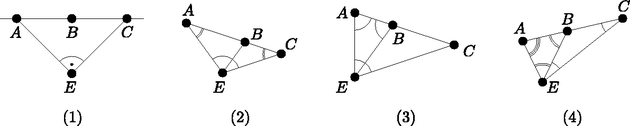We note that configuration $ABCE$ described in $\left(2\right)$ and $\left(4\right)$ can be found (with the same notation) in figure $\left(h\right)$ and $\left(k\right)$ respectively, and configuration $\left(3\right)$ can also be seen in figure $\left(h\right)$, since $DBCE$ and $ABCG$ are both of this type.

Proof. As the sum of angles $EBA$ and $EBC$ is $\pi$, it can be assumed (with the possible change of $A$ and $C$) that the angle at $B$ in triangle $EBC$ is at least a right angle. By Proposition 1, $EB=c$ and $EC>c$. As angle $AEC$ is greater than angles $BEC=BCE$ in triangle $AEC$, $AC>AE$. This triangle is isosceles, and therefore $EA=EC$ or $EC=AC=c+a$.
Let us suppose that $EA=EC$. Then angles $CAE$, $ACE$, $BEC$ are equal and angle $ABE$ is twice as large (since it is the external angle of triangle $BCE$). Thus, the side opposite to vertex $A$ in triangle $ABE$ is shorter than the one opposite to vertex $B$. This triangle is isosceles, and therefore either $a=AB=BE=BC$ (which yields case $\left(1\right)$), or $a=AB=AE$ (then case $\left(2\right)$ is received).
If not $EA=EC$, but $EC=AC=a+c$ holds, then angles $CAE$ and $CEA$ are going to be equal. This latter one is greater than angle $BEA$ and therefore $c=EB>AB=a$ in triangle $ABE$. This is also an isosceles triangle, so either $EA=EB=c$ (then we get case $\left(3\right)$), or $EA=AB=a$ (this gives case $\left(4\right)$). The listed angles can easily be calculated in all of the four cases.  $\square$

Proposition 3. Let us suppose that points $A,D,B,C\in \text{P}$ lie along a straight line $e$ in this order, and $E\in \text{P}$ is an arbitrary point outside $e$. Then $ABCE$ is a configuration described in section $\left(2\right)$ of the former proposition, and $D$ is the reflection of $B$ about the midpoint of $AC$ (see figure $\left(h\right)$). If $\text{P}$ is not collinear, then it can have at most four points along a straight line.

Proof. Let $F$ be the perpendicular projection of $E$ on $e$. According to the previous proposition at most two points along both (closed) half-lines starting at $F$ can belong to $\text{P}$ (since $F$ is an internal point of $AC$ in all configurations described in the previous proposition). Therefore, $F$ is an internal point of $DB$, and both $DBCE$ and $BDAE$ are configurations of type $\left(2\right)$, $\left(3\right)$, or $\left(4\right)$ described in the former proposition. Angle $EBD$ determines the type of both configurations, so the two types are identical (and thus they are reflections of each other about line $EF$). Hence, $EB=ED$, and then both configurations are of type $\left(3\right)$.  $\square$

Corollary 4. Suppose that $\text{P}$ is a $3$-symmetric set that has three collinear points, but is not collinear itself. Then $\text{P}$ is a subset of one of the configurations listed in the theorem.

Proof. Let $A,B,C\in \text{P}$ be points of a straight line $e$ in this order. Let us find all possible points $E\in \text{P}$ outside line $e$.
First, we examine when it is possible to attach two different points $E$ on the same side of $e$ to points $A$, $B$, $C$. The four configurations in Proposition 2 are determined to the extent of similarity. Therefore, if points $A$, $B$, $C$ are given, then point $E$ (on the given side of $e$) is uniquely determined by them. Since Proposition 2 allows swapping $A$ and $C$, in principle one can attach two times four points $E$ to $ABC$.
However, it is not possible to attach a point $E$ to all triplets $ABC$; only to those where the ratio $\frac{AB}{BC}$ is appropriate. This ratio is different in each of the four configurations in Proposition 2. If we are seeking a suitable point $E$ to points $CBA$, then the reciprocal of the above ratio has to be considered. The only possible coincidence is when a configuration of type $\left(2\right)$ is attached to one of the triplets $ABC$ or $CBA$, and a configuration of type $\left(3\right)$ is attached to the other one (on the same side of $e$). This way we get points $E$ and $G$, which can be seen in figure $\left(h\right)$; this is configuration $\left(g\right)$, the only one where there are at least two points on the same side of $e$.
Next, we are going to examine what kind of points $E$ can be on different sides of the line at the same time. The ratio $\frac{AB}{BC}$ determines what type of configurations can be constructed upon $ABC$ or upon $CBA$. Different types can not be confused this time either, except that configuration corresponding to $\left(3\right)$ can be added to configuration corresponding to $\left(2\right)$ on the other side of the line. This is how $\left(f\right)$ in the list of the theorem is obtained ($ABCEU$ in figure $\left(h\right)$). Configurations $\left(1\right)$ and $\left(4\right)$ in Proposition 2 and configuration in Proposition 3 can be reflected about $e$, yielding cases $\left(d\right)$, $\left(k\right)$, and $\left(h\right)$, respectively.
To show that no other configurations exist, we have to prove that if there exists a point in $\text{P}$ on both sides of the line, there can be at most one on each side, namely that there can be no more points on the other side of configuration $\left(g\right)$. As $A$, $E$, $G$ are collinear, propositions stated so far can be applied to these three points instead of $ABC$ (in fact we have swapped $B$ with $E$, and $C$ with $G$). Therefore the side of line $AEG$, which contains $B$ and $C$, can not contain any more points. Since the semiplane determined by the perpendicular line erected on $e$ at $A$, which contains $C$, contains all configurations received so far, $\text{P}$ can not have any more points on the plane, indeed.  $\square$

From now on, let $\text{P}$ be a finite, $3$-symmetric set, which does not have three collinear points. First, we are going to consider the quadrilaterals.

Proposition 5. Let us suppose that $A,B,C,D\in \text{P}$ are four different points. Then configuration $ABCD$ is one of the following:
 $\left(1\right)$ the vertices of an isosceles triangle and the centre of its circumscribed circle;
 $\left(2\right)$ the four vertices of a rhombus;
 $\left(3\right)$ four vertices of a regular pentagon.
If the convex hull of the four points is a triangle, then the first case arises. If it is a quadrilateral, then in cases $\left(1\right)$ and $\left(2\right)$ $ABCD$ is symmetric about the bisectors of all its angles that are at least right angles.

Proof. We are going to use Proposition 1 again. If any of the four points $A$, $B$, $C$, $D$, say $D$ is inside triangle $ABC$, then the sum of the three angles at $D$ ($ADB$, $BDC$, $CDA$) is $2\pi$, and so there can not exist two acute angles among them. But then $DA=DB=DC$ holds, and we are in case $\left(1\right)$.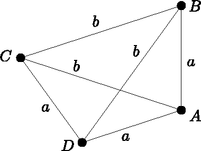Consequently, we can suppose that $ABCD$ is a convex quadrilateral that does not have a vertex which is at equal distance from the other three. As one of the angles of the quadrilateral is at least a right angle, there must be two equal adjacent sides, say $DA=AB=a$. If the quadrilateral is not a rhombus, then e.g. $BC\ne a$ can be assumed, and since three equal sides can not start from $A$, $AC\ne a$. However, triangle $ABC$ is isosceles, and thus $AC=BC=b\ne a$. As three equal sides can not start from $C$ either, $CD\ne b$. One of the sides in triangle $ACD$ is $a$, the other one is $b\ne a$, the third one is not $b$, so it can only be $a$, namely $CD=a$. Therefore, in triangle $DBC$ side $BD$ is also either $a$ or $b$, but it can not be $a$, because no three equal segments can start from $D$, either. So $DB=b$. Calculating the angles we receive a regular pentagon.
Finally, the last proposition for a rhombus is evident. If the configuration consists of the isosceles triangle $ABC$ and the centre $O$ of its circumscribed circle, where $AB=BC$, then because of convexity angle $ABC$ must be obtuse, while from $OA=OB=OC$ it follows that there are acute angles at $A$ and $C$. The angle at $O$ is not necessarily acute; but this is not a problem, as the quadrilateral is symmetric about $OB$.  $\square$

Proposition 6. Set $\text{P}$ is either in a convex position, or all but one of its points are seated along a circle, and the exceptional point is the centre of the circle.

Proof. Let us suppose that point $O\in \text{P}$ lies inside the convex hull of $\text{P}$, and the vertices of the convex hull are ${A}_{1},\text{...},{A}_{n}\in \text{P}$. By induction on $n$, we prove that $O$ is at equal distance from all ${A}_{i}$. If $n=3$, this follows from Proposition 5. Let us suppose that the claim holds for all numbers less than $n$. It is sufficient to prove that $O{A}_{1}=O{A}_{2}$. Let us suppose that this is not so. Then $O$ can not be inside triangle ${A}_{1}{A}_{2}{A}_{3}$ (as the proposition holds for $n=3$). Consequently, it is inside ${A}_{1}{A}_{3}{A}_{4}\text{...}{A}_{n}$, and by the induction hypothesis $O$ is at equal distance from all points ${A}_{1},{A}_{3},{A}_{4},\text{...},{A}_{n}$. This reasoning when repeated for the diagonal ${A}_{2}{A}_{n}$ instead of ${A}_{1}{A}_{3}$ yields that the distance of $O$ from ${A}_{2},\text{...},{A}_{n}$ is the same. But now $O{A}_{1}=O{A}_{n}=O{A}_{2}$. This contradiction proves our statement.  $\square$

Proposition 7. If set $\text{P}$ has at least five points, then it is a subset of the configuration consisting of the vertices and the centre of a regular pentagon.

Proof. Let $A$, $B$, $C$ be adjacent vertices of the convex hull of $\text{P}$, so that angle $ABC$ is at least right angle (there exists such an angle, since, by Proposition 6 the convex hull is at least a quadrilateral). In addition to $A$, $B$, $C$, set $\text{P}$ has at least two more vertices. On the bisector $f$ of angle $ABC$ (as on any straight line) there can be at most two points belonging to $\text{P}$, among which $B$ is one, so there exists $X\in \text{P}$ (and $X\ne A,C$), that is not seated along this bisector. Such a point $X$ can not be the centre $O$ of the circumscribed circle of triangle $ABC$ (because $O$ is on $f$). Hence, by Proposition 6, $ABCX$ is a convex quadrilateral. As $ABC$ is at least a right angle, and $X$ is not on $f$, as a consequence of the last sentence of Proposition 5, $ABCX$ are the four vertices of a regular pentagon. Thus, point $X$ is one of the other two vertices $D$ and $E$ of the regular pentagon containing triangle $ABC$, (i.e. one of $D$ and $E$, say $D$ is in $\text{P}$). By the previous observation, in addition to the vertices of the pentagon, $\text{P}$ can only have a point along $f$ (at most one besides $B$). Let us suppose that $Y$ is such a point. We have to show that $Y=O$. If this is not true, then $O\notin \text{P}$, and hence by Proposition 6 $\text{P}$ is convex, i. e. if $F$ denotes the intersection of diagonals $AD$ and $CE$, then $Y$ can only be seated along the half-line starting from $F$ and not containing $B$. But then $ABY$ is not an isosceles triangle (since $ABCF$ is a rhombus, where $BA=AF because of its angles, and hence both $YA$ and $YB$ are greater than $AB$). With this we have proved both the proposition and the theorem itself.  $\square$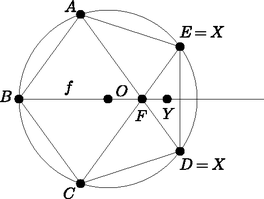Generalizations

The question of what can be said about those planar point sets, all of whose four-element subsets are axially symmetric comes naturally. Or, in general, one can ask, that for a given number $k$ which point sets will be $k$-symmetric, which means that any $k$-element subset of them is axially symmetric.
Next come some examples of non-collinear, $k$-symmetric sets, that ‐ by arbitrary $k$ ‐ can be found by anyone after some investigation. It is plausible that the best way to find such sets is not to keep $k$ fixed, but to first require the set to remain axially symmetric after leaving any point off from the set. Let these sets be called one-less-symmetric.
It can be easily seen that the following planar point sets are all one-less-symmetric:
 $\left(a\right)$ all vertices of a regular polygon;
 $\left(a\text{'}\right)$ all vertices and the centre of a regular polygon;
 $\left(b\right)$ all but one vertices of a regular polygon;
 $\left(b\text{'}\right)$ all but one vertices and the centre of a regular polygon;
 $\left(c\right)$ points along two perpendicular straight lines that are axially symmetric about both lines;
 $\left(d\right)$ the union of the vertices of some concentric (and different sized) regular $m$-gons and $2m$-gons, so that the axes of symmetry of the $m$-gons coincide (i.e. $m$-gons can have two different positions), and the vertices of the $2m$-gons are seated along the axes of symmetry of the $m$-gons (and so the $2m$-gons can only have one position);
 $\left(d\text{'}\right)$ a system described in $\left(d\right)$ united with the common centre of the polygons.

It can be noticed that point sets listed in $\left(a\right)$ and $\left(a\text{'}\right)$ are also two-less-symmetric, i. e. leaving off any two points, the remaining system is axially symmetric.
Are there any more finite, non-collinear, one-less-symmetric point sets in the plane, other than those listed above? It can be proved that actually there are not. We are going to leave the solution of this problem to the reader, adding that there are no more two-less-symmetric sets apart from those listed above, either.

Problems:
 $\left(1\right)$ Prove that any finite, one-less-symmetric subset of the plane that has at least five elements, is one of the examples $\left(a\right)$‐$\left(d\text{'}\right)$.
 $\left(2\right)$ Prove that any finite, two-less-symmetric subset of the plane that has at least six elements, is one of the examples $\left(a\right)$ and $\left(a\text{'}\right)$.

Using these results, a full overview can be obtained on $k$-symmetric point sets of the plane for all $k$. For instance, it easily follows that for $k\ge 4$, if a $k$-symmetric point set has at least $k+3$ elements, then it is collinear.
Eventually, it is an interesting question that relaxing finiteness while looking for one-less- or two-less-symmetric sets, what other examples arise. (Such sets are for example sets comprising all points of a circle or a circular disk, or sets consisting of all points with rational coordinates, and a lot of other examples that can be constructed similarly.) The investigation and description of these sets will also be left to the reader.
1Supported by OTKA T043671.

2Supported by OTKA T032478.